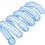# An Accidental Hint at Euler's Equation

I had this thought at lunch today. It's not a sophisticated treatment of the subject, but I found it amusing.

We know that when you differentiate a sinusoid twice, you get back a scaled and negated version of the original. Here the scaling factor is unity.

$y = sin(t) \\ \ddot{y} = -sin(t) = -y$

We also know that double-differentiating an exponential gets us back a scaled (but not negated) version of the original. Here again, the scaling factor is unity.

$y = e^t \\ \ddot{y} = e^t = y$

These two behaviors are tantalizingly similar. So how might we get the exponential to behave like the sinusoid with respect to double-differentiation? Maybe we could throw in the square root of negative one.

$y = e^{j t} \\ \ddot{y} = j^2 e^{j t} = -e^{j t} = -y$

Making the exponent complex makes the exponential behave like a sinusoid with respect to double-differentiation. Hence, we've stumbled onto something like Euler's equation (shown below for reference).

$e^{j t } = cos \, t + j \, sin \, t$Note by Steven Chase
3 years, 1 month ago

This discussion board is a place to discuss our Daily Challenges and the math and science related to those challenges. Explanations are more than just a solution — they should explain the steps and thinking strategies that you used to obtain the solution. Comments should further the discussion of math and science.

When posting on Brilliant:

• Use the emojis to react to an explanation, whether you're congratulating a job well done , or just really confused .
• Ask specific questions about the challenge or the steps in somebody's explanation. Well-posed questions can add a lot to the discussion, but posting "I don't understand!" doesn't help anyone.
• Try to contribute something new to the discussion, whether it is an extension, generalization or other idea related to the challenge.

MarkdownAppears as
*italics* or _italics_ italics
**bold** or __bold__ bold
- bulleted- list
• bulleted
• list
1. numbered2. list
1. numbered
2. list
Note: you must add a full line of space before and after lists for them to show up correctly
paragraph 1paragraph 2

paragraph 1

paragraph 2

[example link](https://brilliant.org)example link
> This is a quote
This is a quote
    # I indented these lines
# 4 spaces, and now they show
# up as a code block.

print "hello world"
# I indented these lines
# 4 spaces, and now they show
# up as a code block.

print "hello world"
MathAppears as
Remember to wrap math in $$ ... $$ or $ ... $ to ensure proper formatting.
2 \times 3 $2 \times 3$
2^{34} $2^{34}$
a_{i-1} $a_{i-1}$
\frac{2}{3} $\frac{2}{3}$
\sqrt{2} $\sqrt{2}$
\sum_{i=1}^3 $\sum_{i=1}^3$
\sin \theta $\sin \theta$
\boxed{123} $\boxed{123}$

Sort by:

Good Lord. Thanks sir for posting these.

- 3 years, 1 month ago

- 3 years, 1 month ago

Very interesting. Great work.

- 2 years, 9 months ago

Thanks

- 2 years, 9 months ago